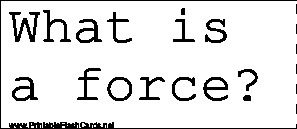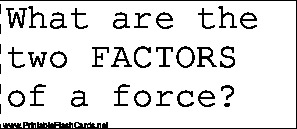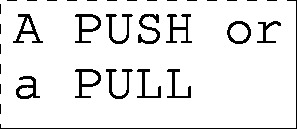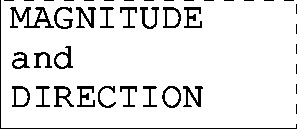Sample flash cards in this set:

Front:Back:This set includes the following cards:
What is a force?A PUSH or a PULL
What are the two FACTORS of a force?MAGNITUDE and DIRECTION
Objects will ______ when acted upon by an unbalanced force.ACCELERATE (speed up, slow down, change direction)
When an object is subject to 2 or more forces at once, the result is a _____ _____.NET FORCE
If two forces of EQUAL magnitudes act in OPPOSITE directions, what would be the net force?0 NEWTONS
______ is the tendency of an object to resist a change in its motion.INERTIA
______ is the amount of matter in an object.MASS
If the mass of an object is INCREASED, the inertia of the object will _______.INCREASE
_____ is a force created by one object rubbing against the surface of another object.FRICTION
What types of friction will we talk about in science class?ROLLING, FLUID, and SLIDING FRICTION
What is the term for the force that holds everything in its place in the universe?GRAVITY
What is the term for the force that pulls objects toward the center of the planet they are on?GRAVITY
If 2 forces of UNEQUAL magnitudes act in OPPOSITE directions, the net force's DIRECTION would be the same as the ______ force.LARGER FORCE
_____ _____ is used for the end result of forces combining together.NET FORCE
Newton's 1st law of Motion tells us that an object that is at rest will REMAIN at REST, or if its moving, it will CONTINUE to MOVE, unless acted upon by an _____ _____.UNBALANCED FORCE
_______ is the "stuff" that makes up everything in the universe.MATTER
______ is the unit of measurement for force.NEWTON
How many Newtons does a balanced force have?0 NEWTONS
What two things are needed for an object to have weight?MASS and GRAVITY